DIY

# Magnetic Levitation with Solenoid Coil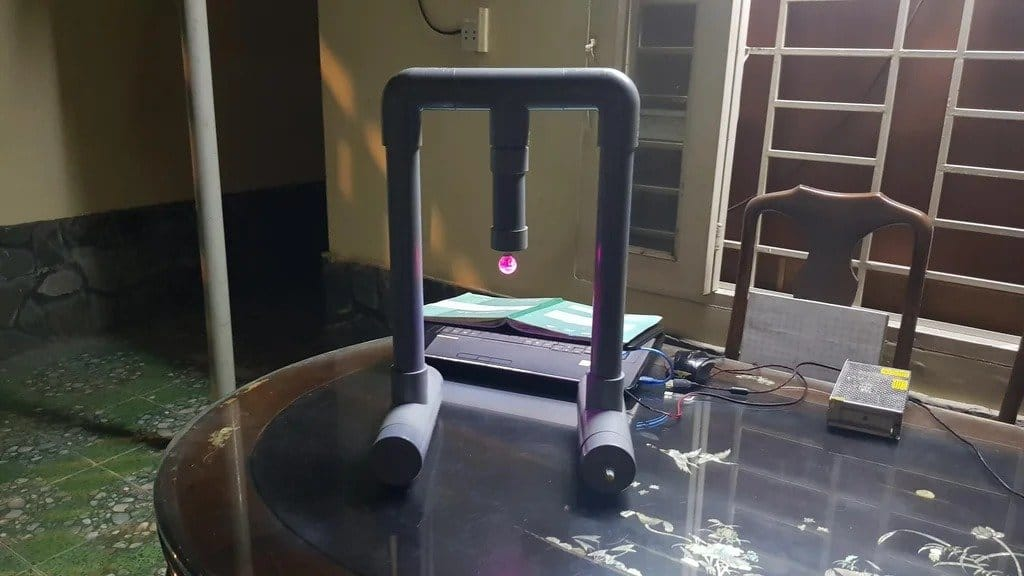In the article “Globe Levitating in a Magnetic Field” told about an interesting experiment on levitating an object in a magnetic field. The article aroused a certain interest and discussion, which then moved to the article by the DIYer ino53 Based on of the publication “Globe Levitating in a Magnetic Field” .
Continuing the topic of magnetic levitation, today we will consider an article by the DIY master Tuenhidiy. In the article, he presents his device for levitating small objects. For clarity, he uses as a “levitating” object, a child's toy – a glowing ball.
Let's watch a small demo video.

Of course, this device is not as spectacular as a globe, but it is also easier to manufacture.
Tools and materials: -Inductance coil; -Arduino Nano; -KY-024 linear magnetic Hall sensor;
-NPN TO-220 or TIP122 transistor;
-Resistor 10K; -N4007 diode; -Printing board 5X7 cm; – Screw connector – 2 pcs; – DC power plug + connector – 2 pcs; – DC power supply 5 V and 12 V; -Tape cable 8 and 16 wires; -Power cable; -Neodymium magnets;
-PVC corner pipe Ø 42 mm – 2 pieces;
-PVC pipe Ø42 mm – 1 meter;
-PVC tee Ø 42 mm;
-PVC pipe plugs Ø 42 mm – 2 pcs;
-Transitional tee from Ø60mm to Ø42mm – 2 pcs;
-Cap of PVC pipes Ø 60 mm – 4 pcs;
-Drilling machine; -Haw; -Soldering accessories;
Step one: scheme
Scheme of the project is presented in the sketch.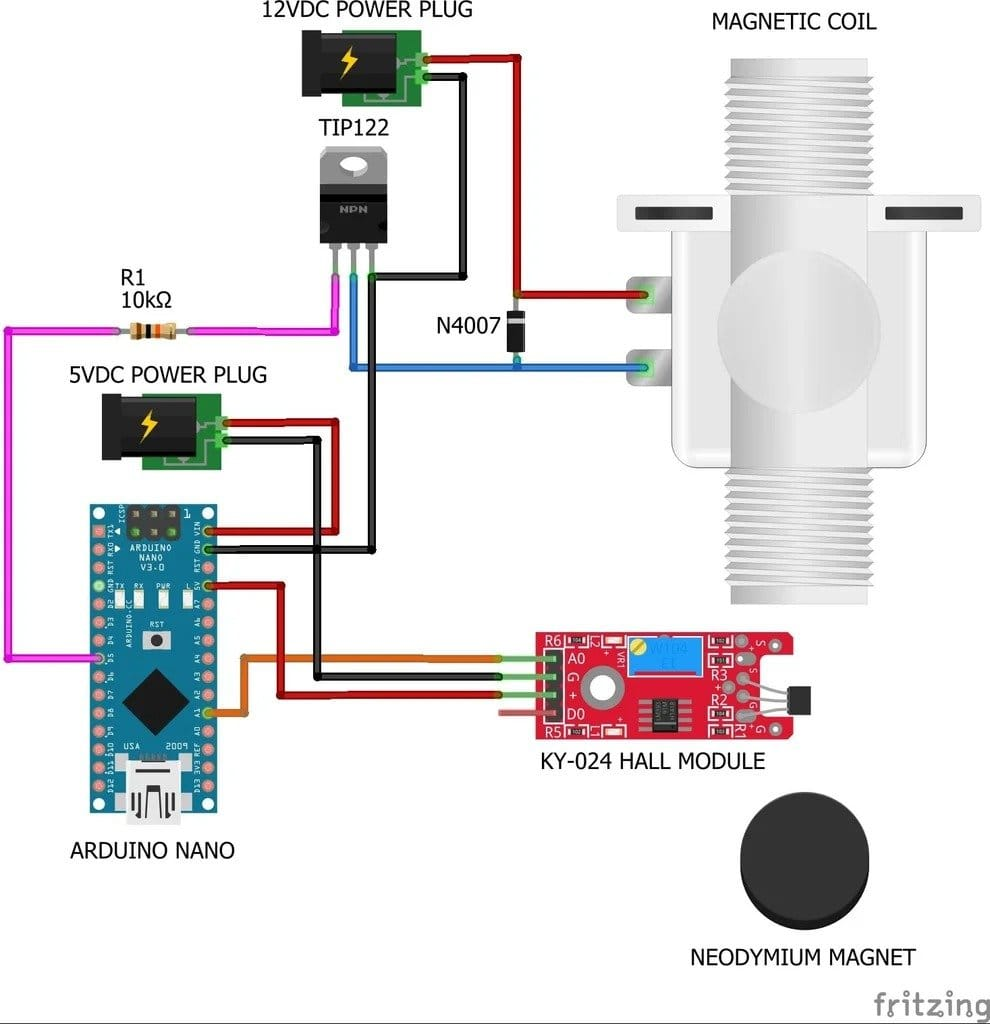Step Two: Selecting the Hall Module
There are many magnetic sensor modules sold on the market, and as described, they can output both digital and analog signals, but this is not the case. The master has purchased several modules, and they only have a digital signal. In the end, he chose the KY-024 module.
The KY-024 linear magnetic Hall sensor reacts to the presence of a magnetic field. It has a potentiometer for adjusting the sensitivity of the sensor and provides both analog and digital outputs. This module consists of a 49E linear Hall effect sensor, an LM393 dual differential comparator, a potentiometer, LEDs and resistors.
49E is a linear Hall effect sensor. It can measure both north and south polarity of the magnetic field, and the relative strength of the field. It works like this:
If there is no magnetic field, the 49E will output a voltage of about half the source voltage, about 2.5 V.
If the south pole of the magnet is near the marked side 49E, then the output voltage will increase linearly towards the source voltage (up to about 4.2 V).
If the north pole of the magnet is near the marked side 49E, then the output voltage will be linear fall relative to the strength of the magnetic field (drops to about 0.86 V).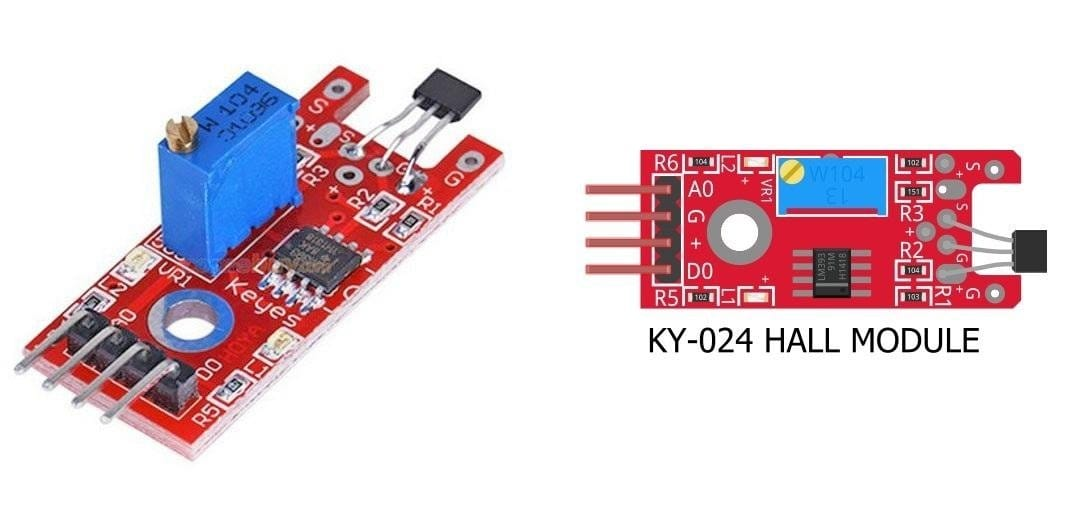Step Three: Magnetic Coil
This magnetic coil is removed from the magnetic lock. The craftsman removed the metal body and core, leaving only the coil. Its operating voltage is 24 VDC.
Using a multimeter, he measured the resistance of the coil, it is 15.0 ohms. In this project, the master uses 12 VDC to power this coil.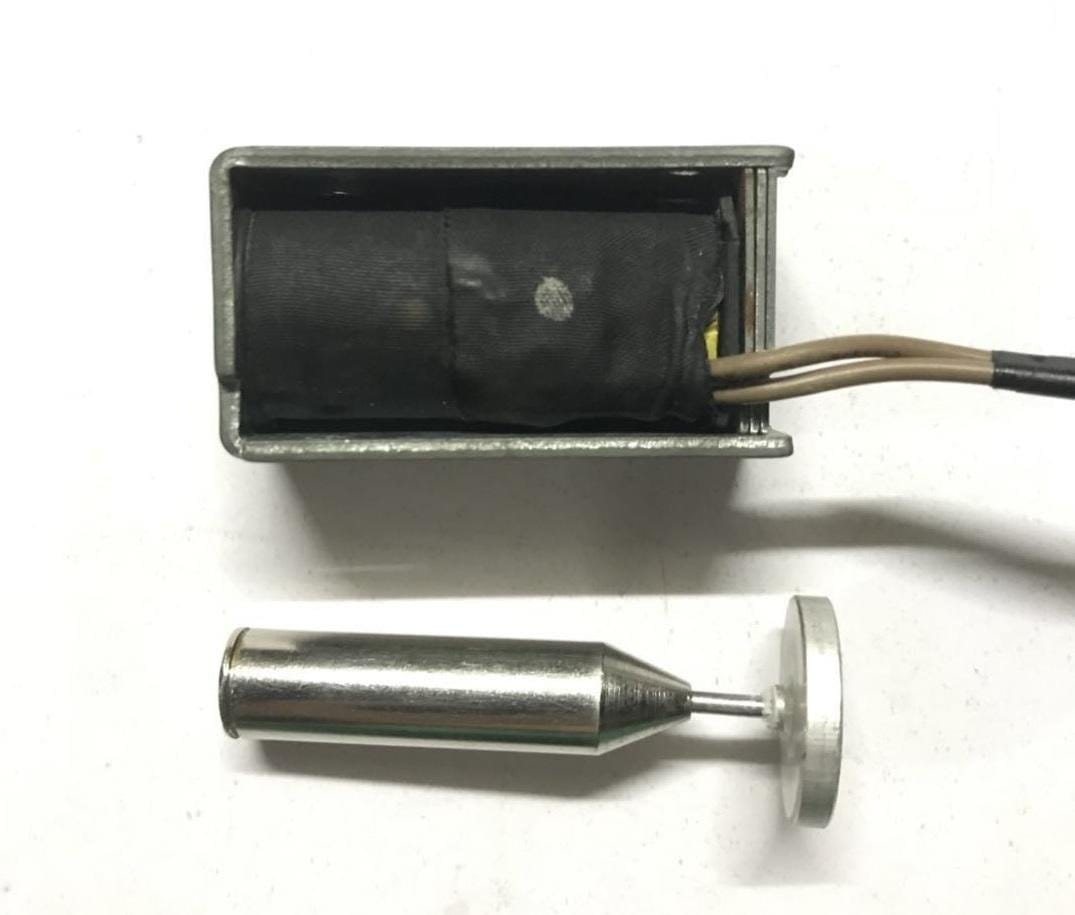Step four : preparation of parts
First you need to prepare the Hall module. Dismantle Hall element 49E from the board. The wires are soldered in place of the element.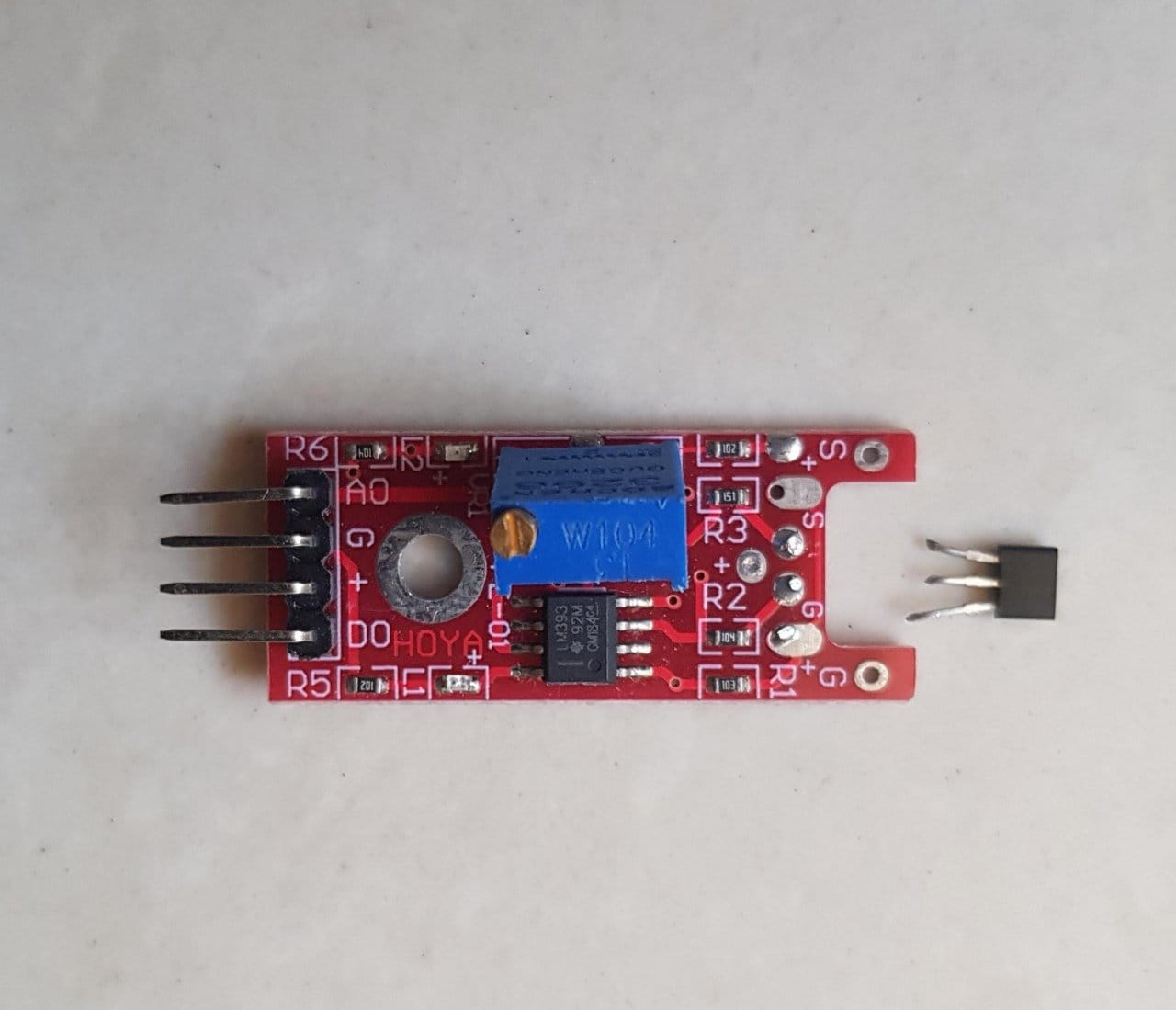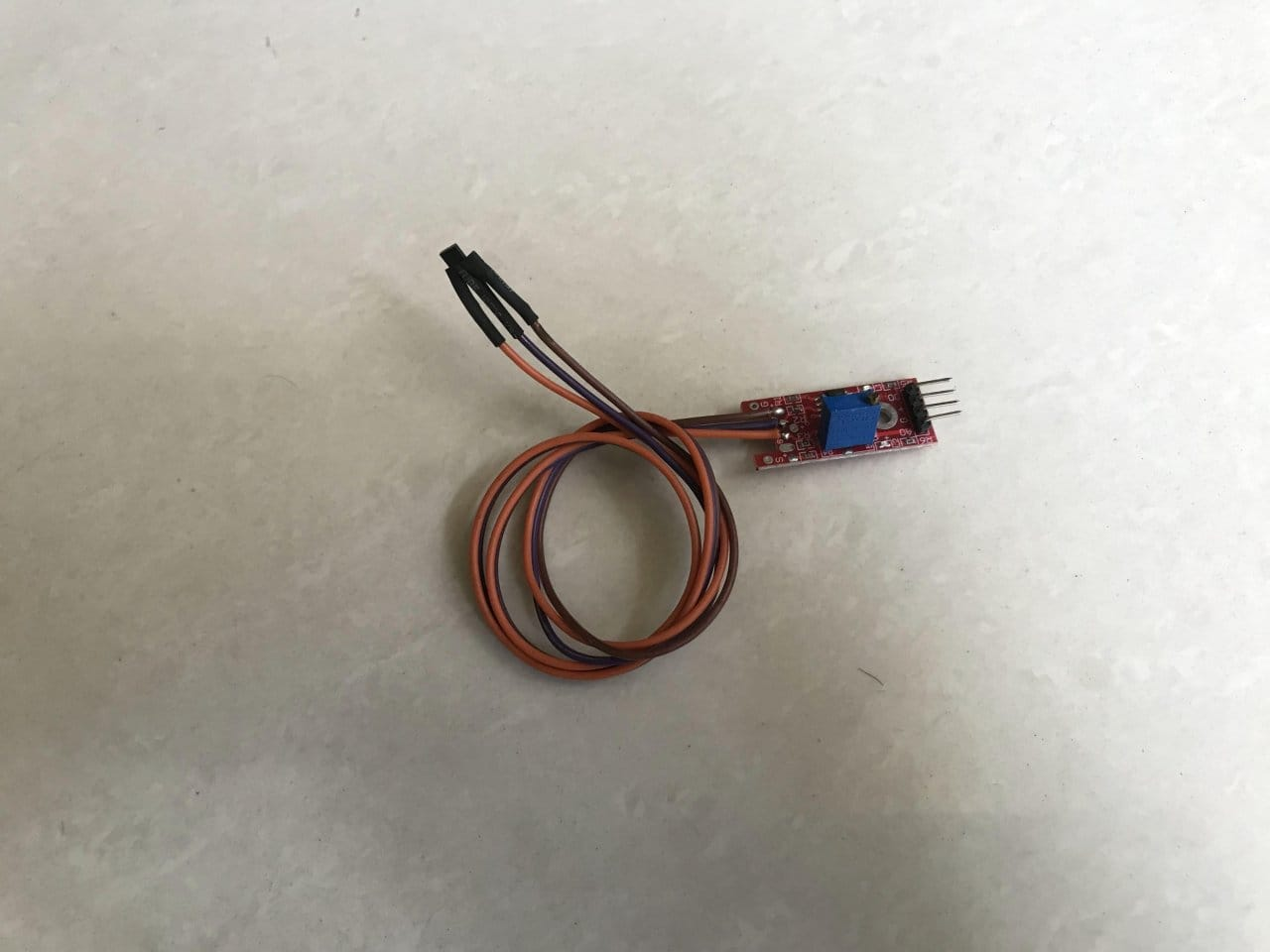Now you need to mount the board.
According to the diagram, he soldered all the connections and components on the prototype printed circuit board 50×70 mm.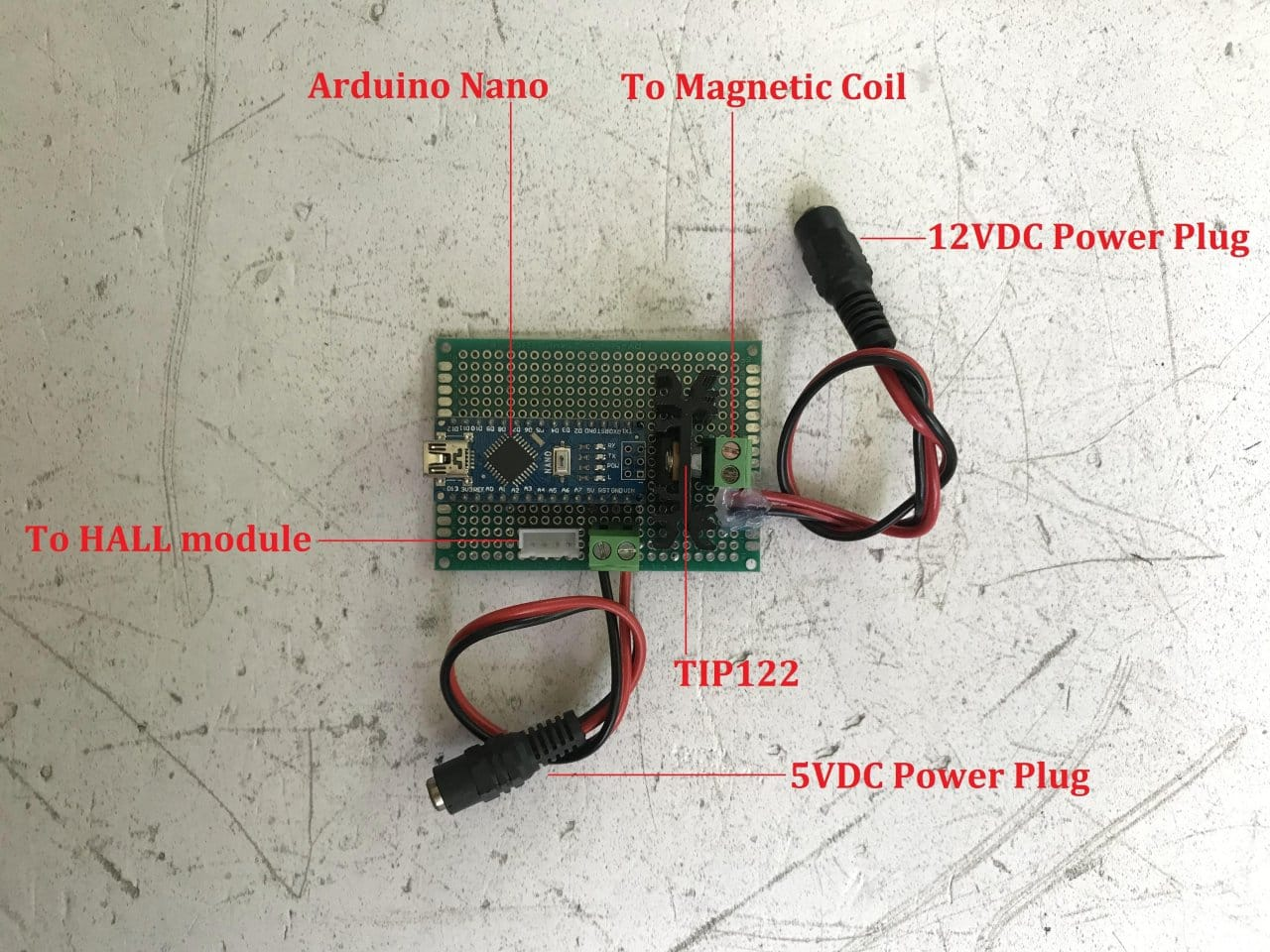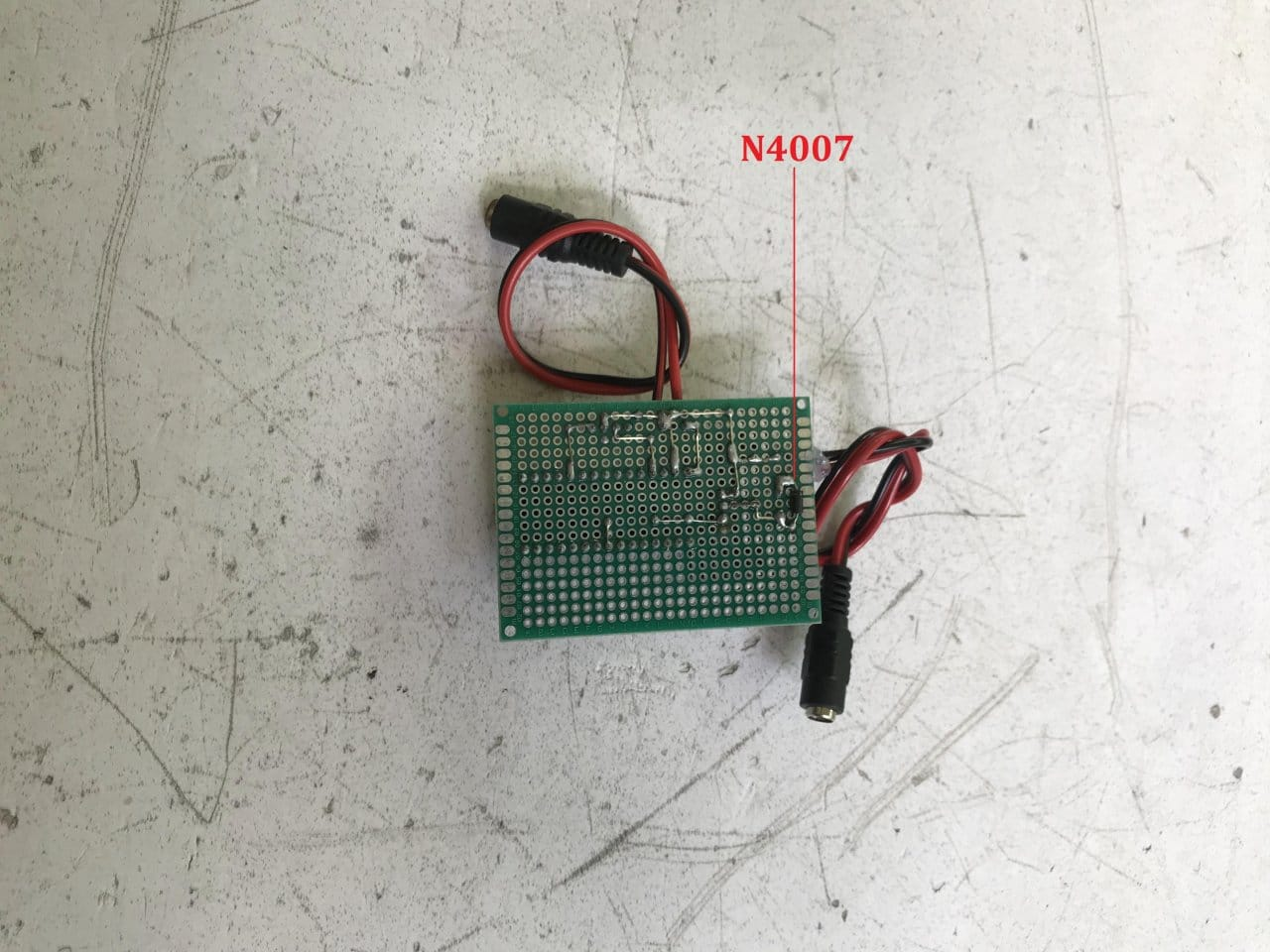Step four: determining the polarity of the magnetic coil and neodymium magnets
Before assembly, the polarity of the magnetic coil and neodymium magnets must be determined.
The wizard used a simple program to read the analog value from the Hall sensor module.

` ` int HallValue = 0; void setup () & # 123; Serial.begin (9600); } void loop () & # 123; HallValue = analogRead (A1); Serial.println (HallValue); } ` `

Or you can use a multimeter to measure the output voltage of the sensor.
Then he connected the magnetic coil to a 12V DC power supply and the Hall module to the Arduino Nano. Places the two ends of the coil alternately next to the 49E Hall sensor and reads the Hall values ​​on the Arduino IDE serial monitor.
If there is no magnetic field, the value is around 512.
If the read value increases from 512, it is the south pole.
If the reading drops to 512, this is the North Pole.
He also determined the polarity of the magnets and marked them.
Step Five: Assembly
Now we need to assemble the rack and the device.
First assembles the upper part of the device.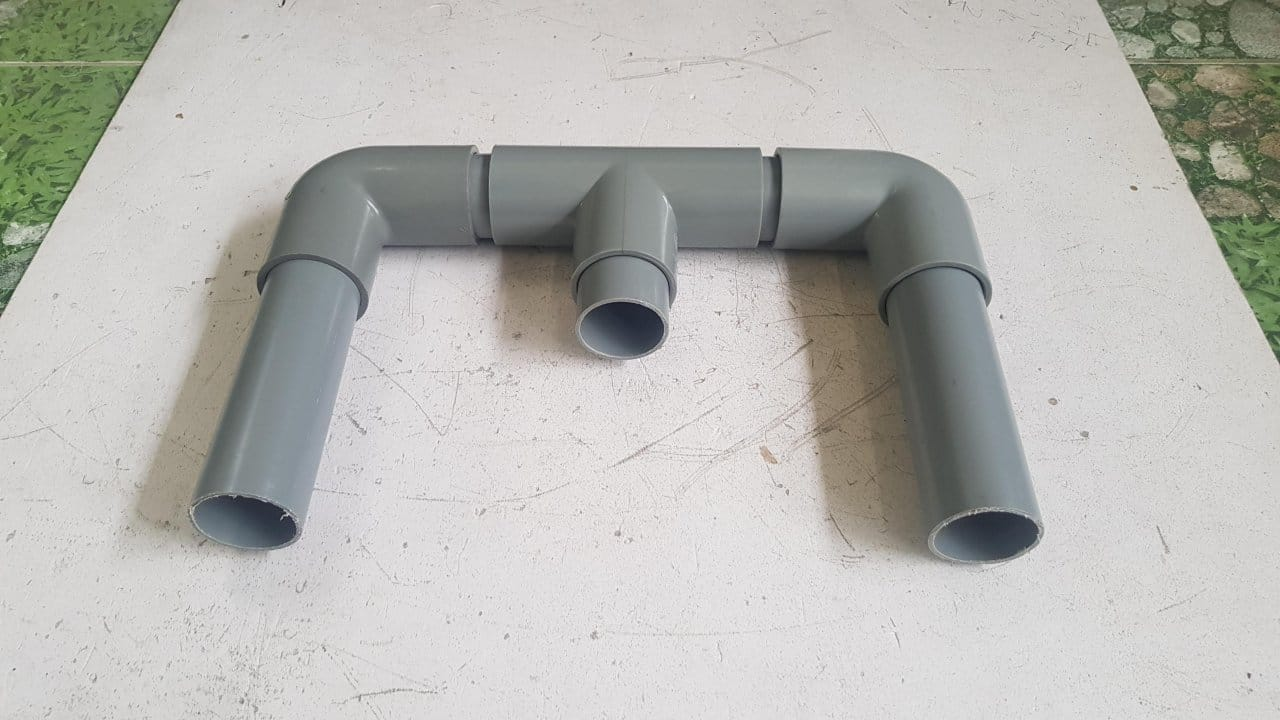Drill a hole in the plug and screw the fitting.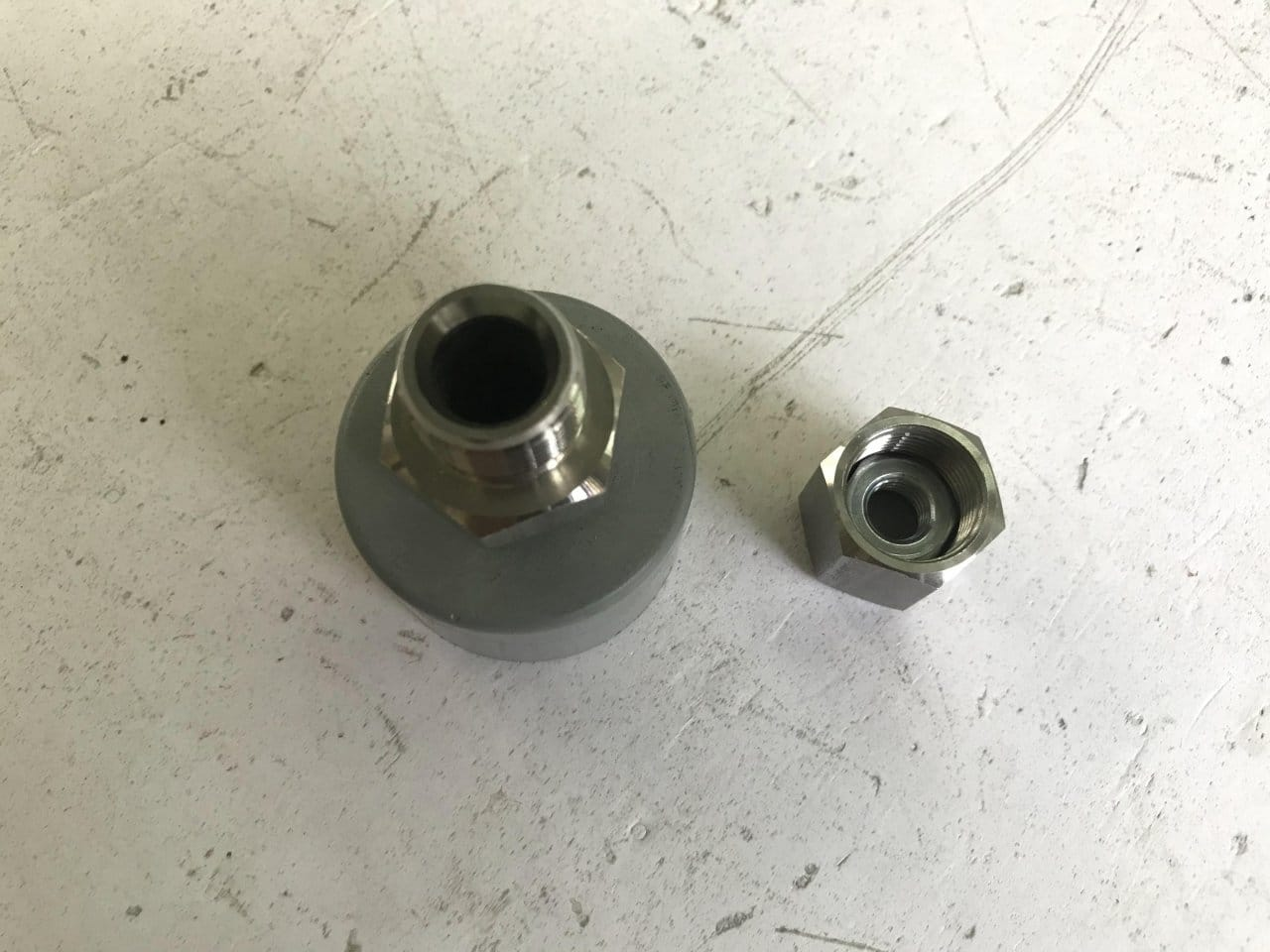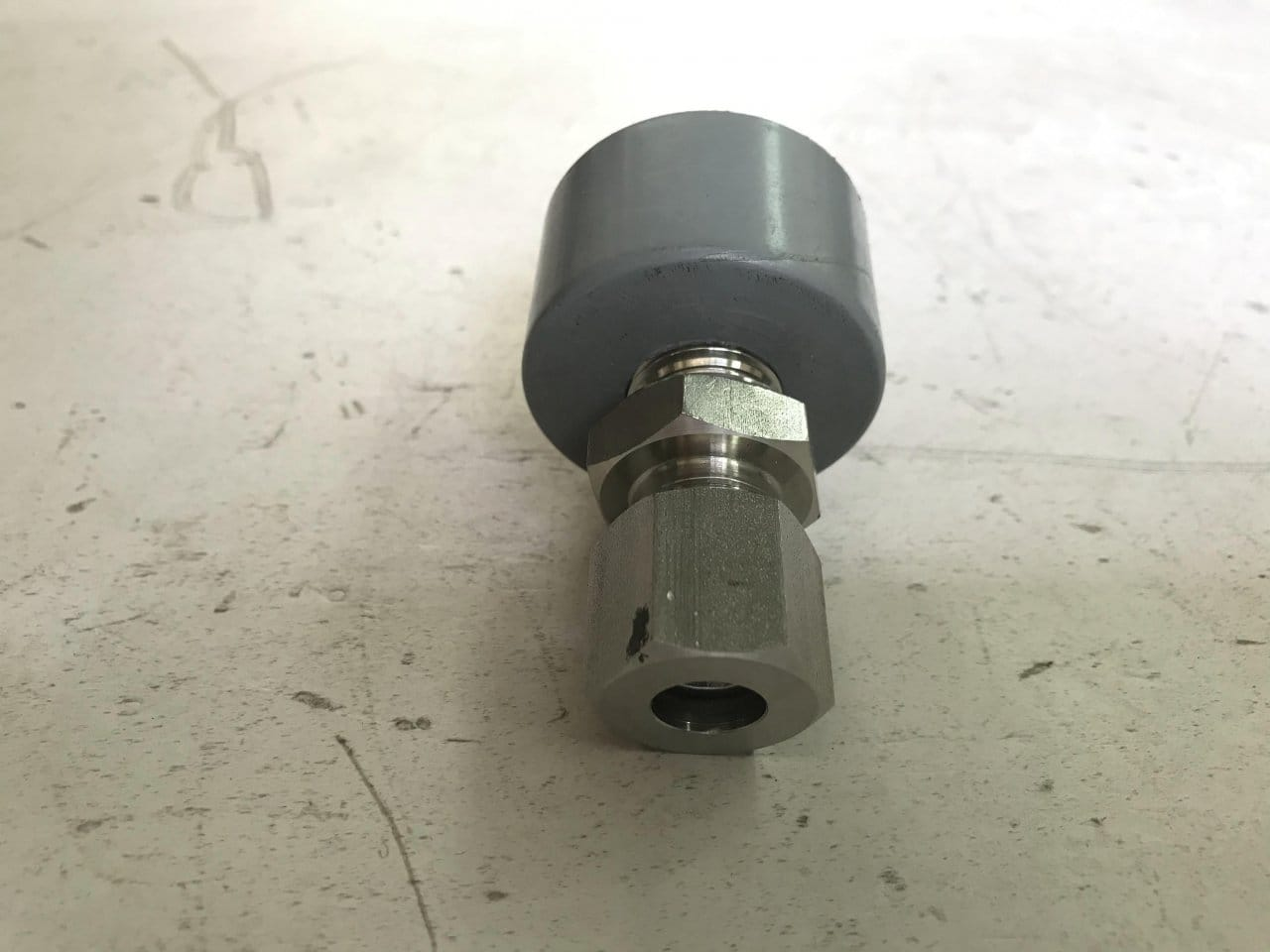Screws a hairpin into the fitting and cut it to the desired length.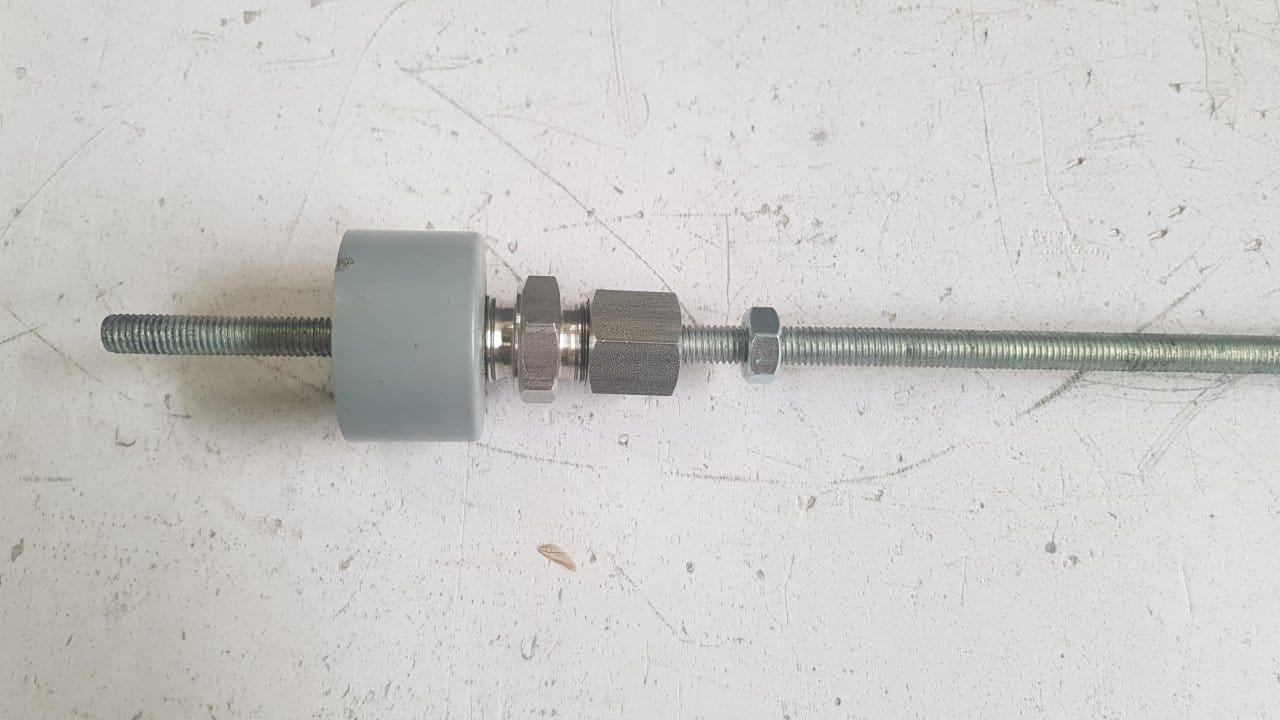Installs the coil … The polarity must be determined as in the photo.He then glued one M10 nut on top of the magnetic coil. This way you can adjust the length of the suspension bolt, which is in the magnetic coil.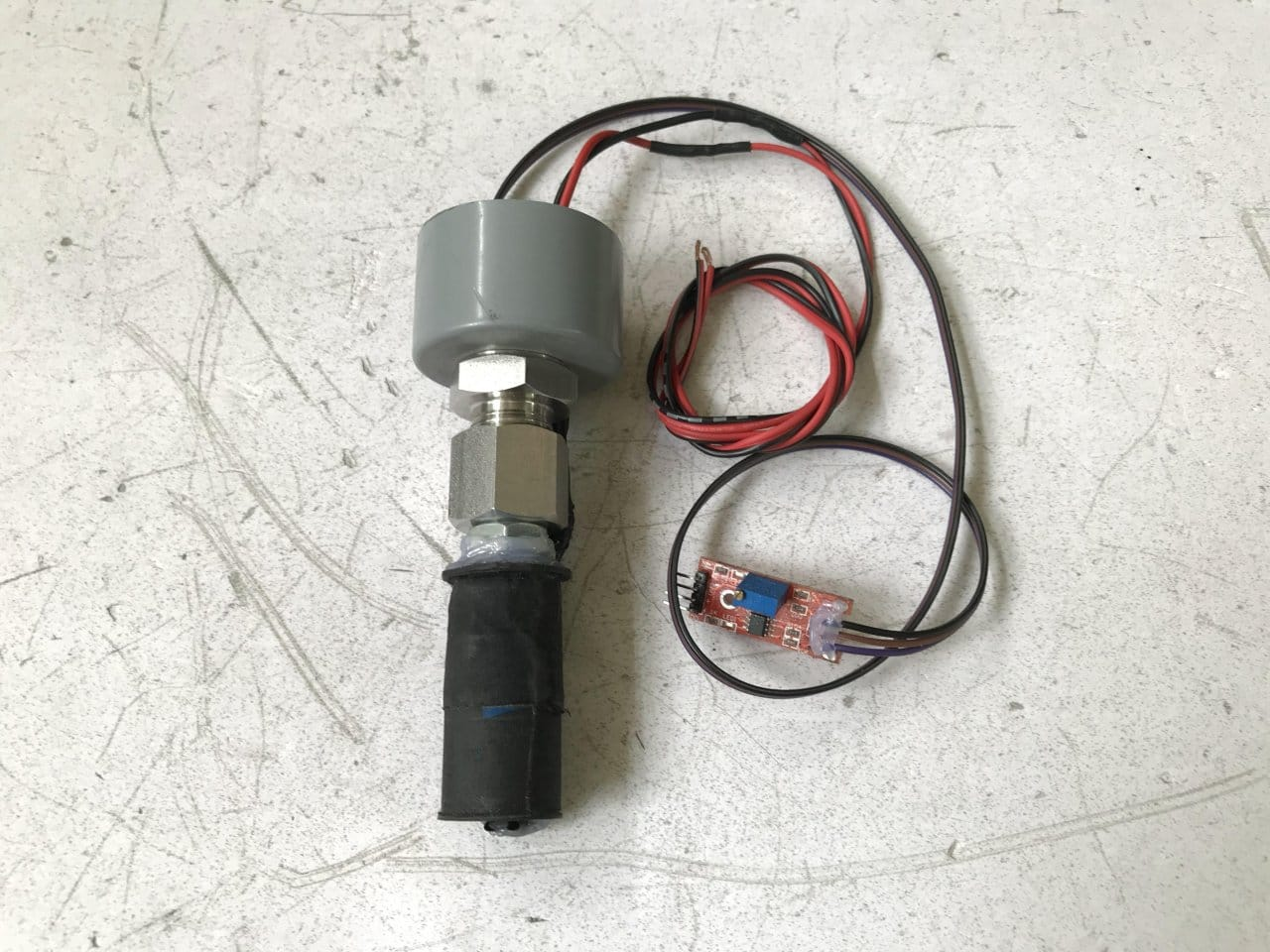The Hall sensor was also glued to the bottom of the coil with the inscription down. As you can see in the picture, the steel core was adjusted with a gap of about 5 mm with a Hall sensor.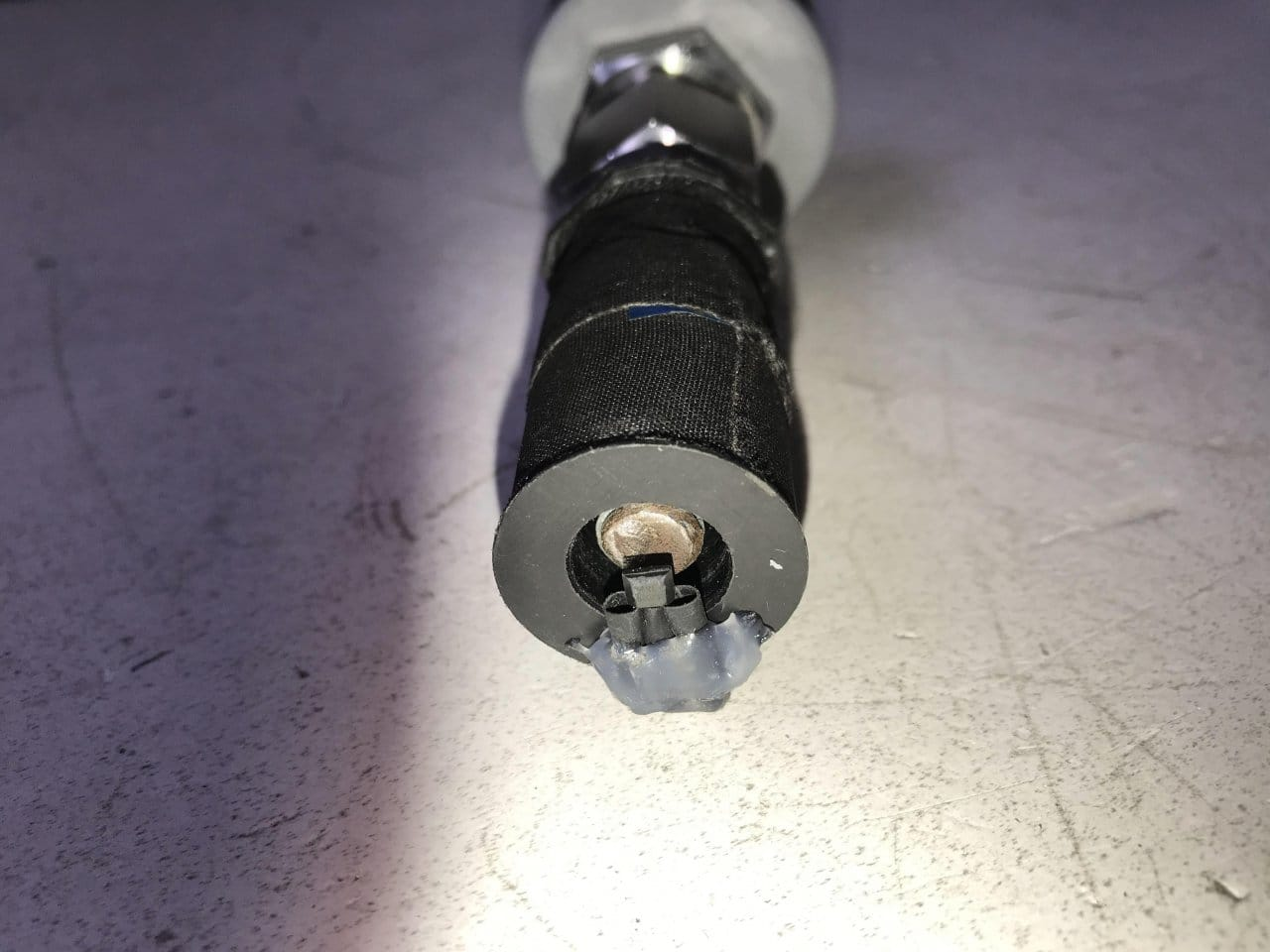The magnetic coil must be installed in the section from the PVC pipe and closed with a lid. He cut a window in the pipe so that the magnetic coil could dissipate heat.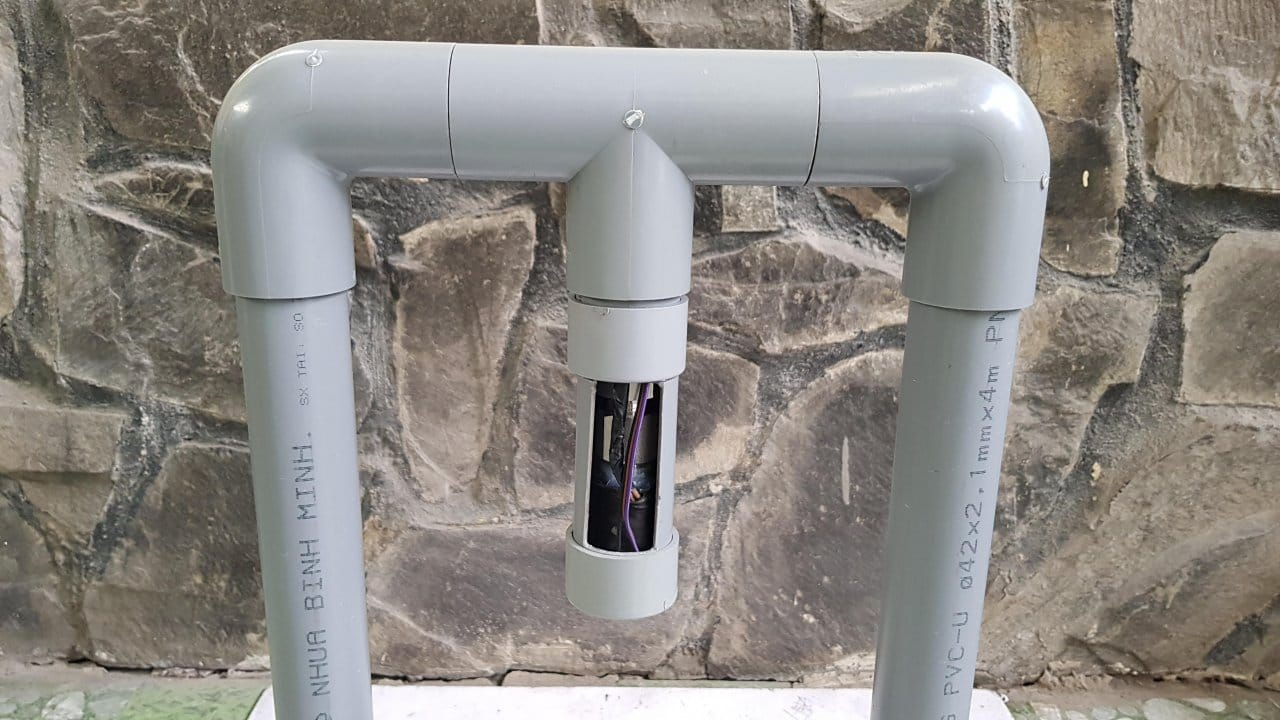Two transition tees from Ø60mm to Ø42mm were used as the base of the levitator. They also contain a control board and a KY-024 module. All cables were run inside the pipe frame.
He installed two power plugs (12 and 5 VDC) on 60mm PVC end caps and drilled one hole for the Arduino programming cable. All of them are located on the back of the levitator. Installs a potentiometer on the front side.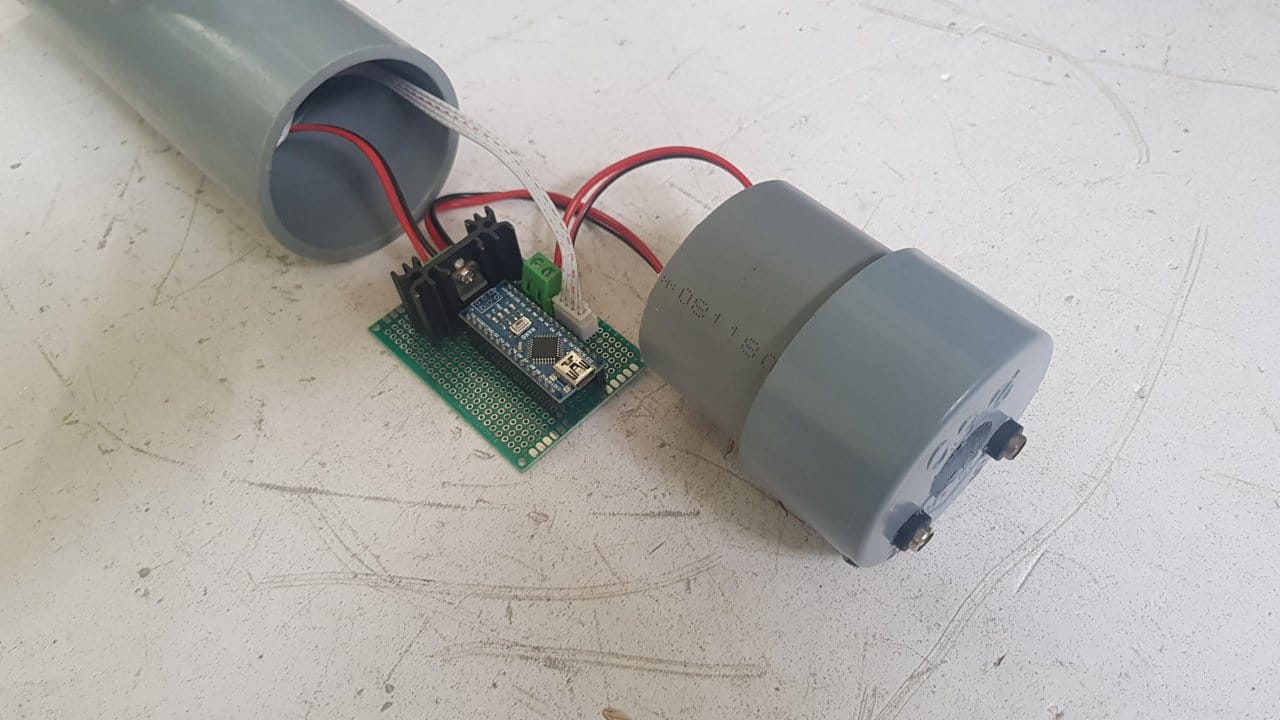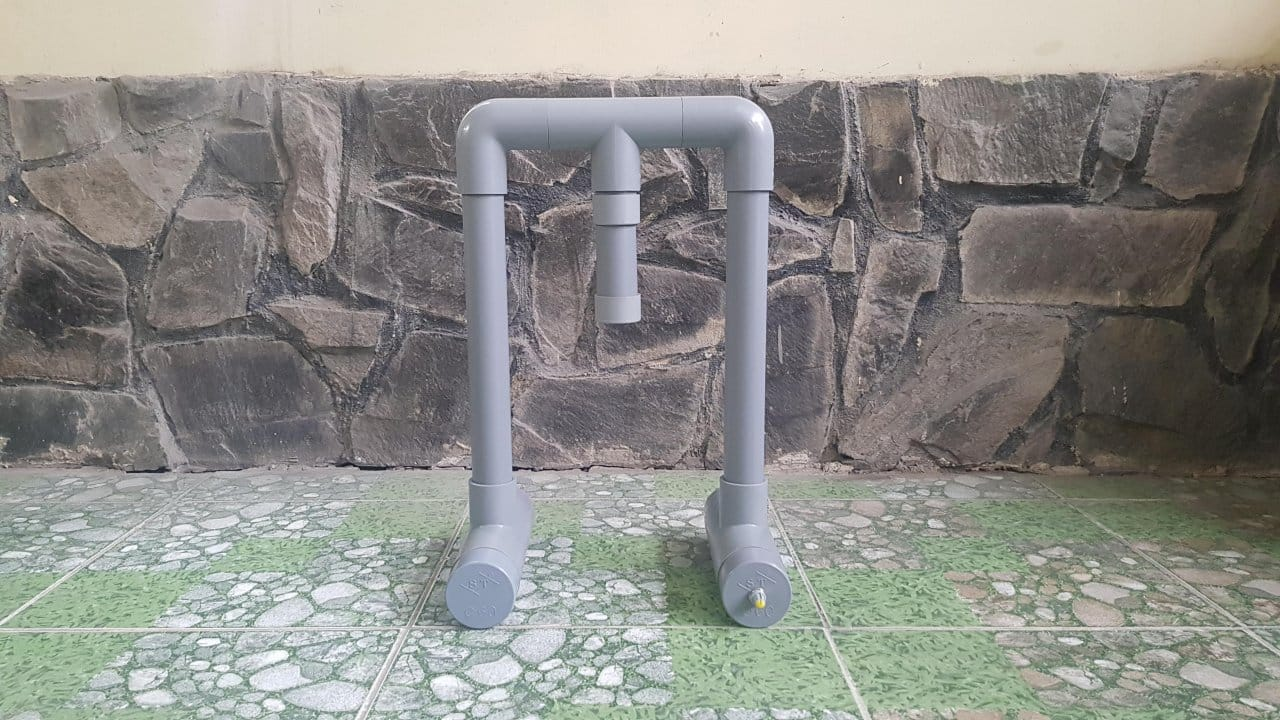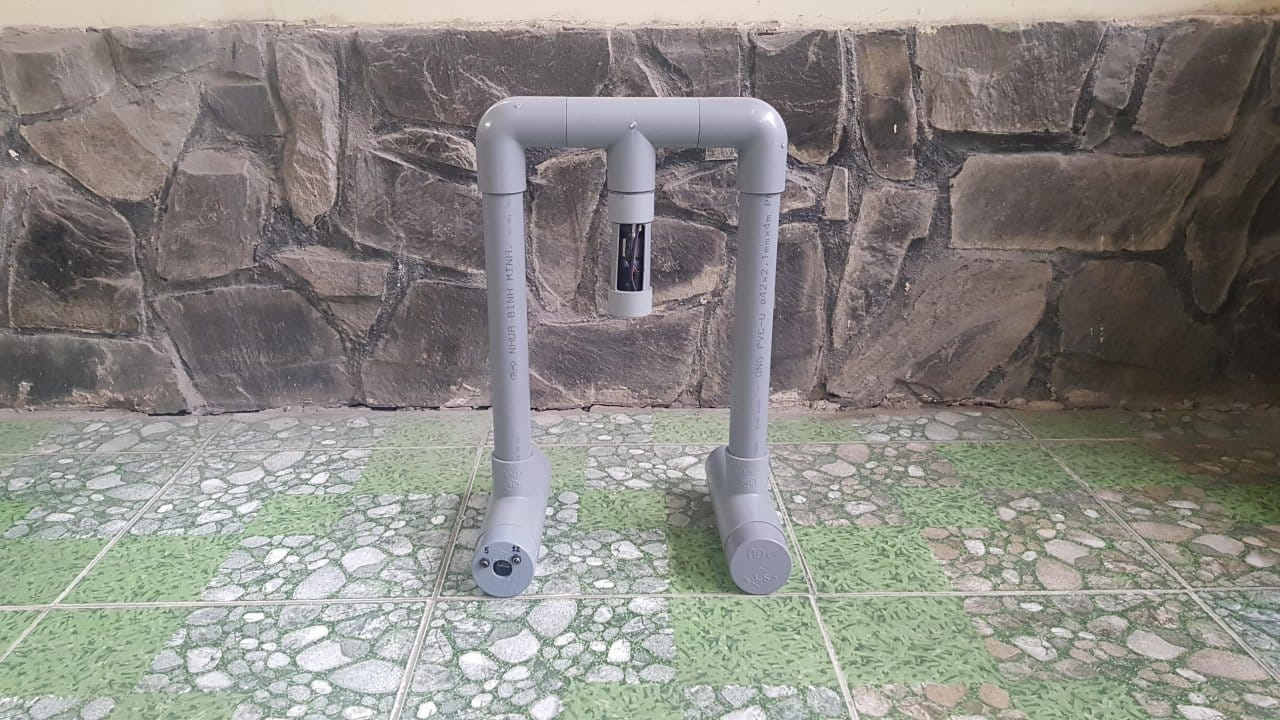Step six: programming

` `#define outMin 0 //Low limit for P.I.D output #define outMax 255 //High limit for P.I.D output #define Hall_Input_Pin A1 //Arduino Pin A1 - Read analog value from Hall Module. #define Coil_Output_Pin 5 //Arduino Pin D5 - Control magnetic coil. #define DEBUG 1 //Turn ON/OFF debugging //PID parameteres int Hall_Input = 0; int Coil_Output = 0; double Setpoint = 330.0; double Input = 0.0; double Previous_Input = 0.0; double Output = 0.0; double Integral = 0.0; double Derivative = 0.0; double Error = 0.0; double dInput = 0.0; double timeChange = 0.1; double KP = 0.6; double KI = 0.4; double KD = 0.01; unsigned long millisecond = 0; void setup () & # 123; Serial.begin (115200); pinMode (Coil_Output_Pin, OUTPUT); } void loop () & # 123; //Read Input - Hall Sensor Hall_Input = analogRead (Hall_Input_Pin); Input = Hall_Input; //Calculate the Error Error = Setpoint - Input; dInput = (Input - Previous_Input); //PID Algorithm Integral + = Error * timeChange; Derivative = dInput/timeChange; Output + = - KP * Error - KI * Integral + KD * Derivative; //Write to Ouput if (Output & gt; outMax) Output = outMax; if (Output & lt; outMin) Output = outMin; Coil_Output = Output; analogWrite (Coil_Output_Pin, Coil_Output); //Remember the previous Input Previous_Input = Input; //Show debugging values ​​if (DEBUG) & # 123; if ((millis () - millisecond) & gt; 500) & # 123; //Show the Setpoint Serial.print (Setpoint); Serial.print (& # 34;/& # 34;); //Show the Input Serial.print (Input); Serial.print (& # 34;/& # 34;); //Show the Error Serial.print (Error); Serial.print (& # 34;/& # 34;); //Show the Output; Serial.print (Output); Serial.println (& # 34 ;; & # 34;); millisecond = millis (); }}} ` `

Step Seven: Calibration
The PID process is divided into two groups: direct and reverse acting. Magnetic Levitation is a reverse acting PID controller. This means that its output tends to decrease as the measured signal (input) increases.
In the PID formula, instead of adding (Kd * derivative of error), we subtract (Kd * derivative of the input data) because the levitation setting (in this case) is constant (330).

` ` Output + = - KP * Error - KI * Integral + KD * Derivative; ` `

The derivative part is calculated as follows:

` ` dInput = (Input - Previous_Input) ; Derivative = dInput/timeChange; ` `

The wizard has manually calibrated the levitator and the final PID parameters are as follows:

` `double timeChange = 0.1; double KP = 0.6; double KI = 0.4; double KD = 0.01; ` `

To fine tune and see how the levitator works, you need to enable debug mode and read data from the IDE serial port.The diagram below shows the operation of the reverse acting PID. The wizard used the Pivot function in Excel to generate a graph from the IDE's serial data.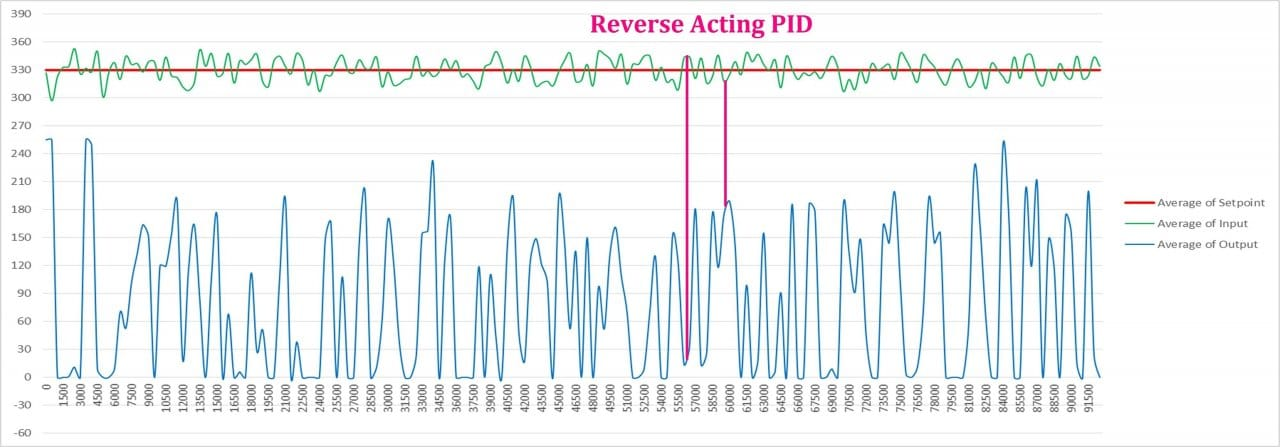Diagram abstract :
Red line – Setpoint (in this case 330).
Green curve – Input (feedback from the Hall sensor).
Blue curve – Output (PWM signal to the magnetic coil).
For correct operation of the device, the following settings must be made and the following conditions must be observed:
It is necessary to adjust the potentiometer on the KY-24 module for sensitivity.
It is necessary to adjust the length of the pin, which is located inside the magnetic coil.
Calibration of the setpoint and PID coefficients.
Selecting the correct floating loads plus the weight of the magnet. If it is too light or too heavy, the levitator will not work. Finally, the cargo must be symmetrical.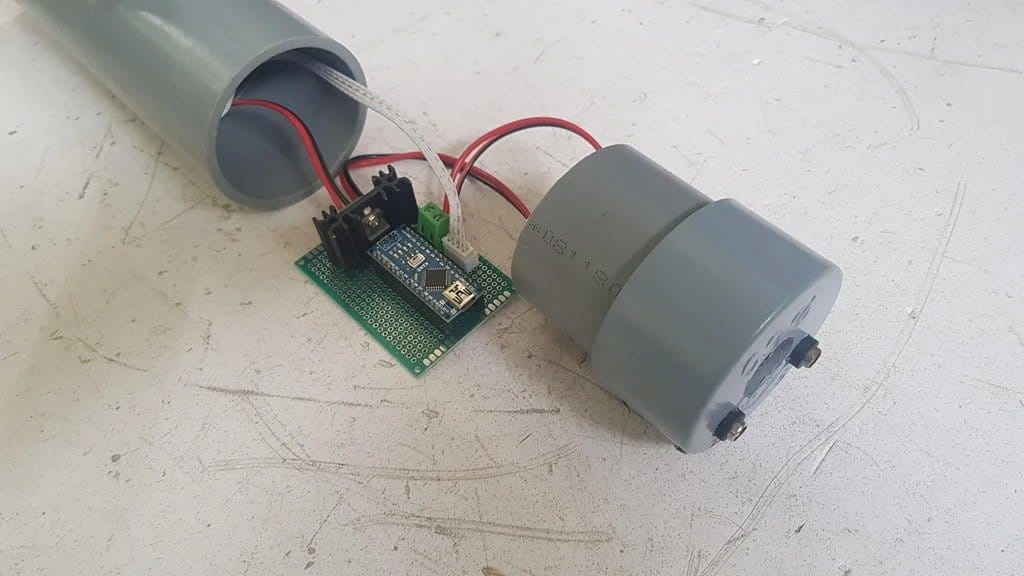As the master used a ball with an LED inside to liven up the object.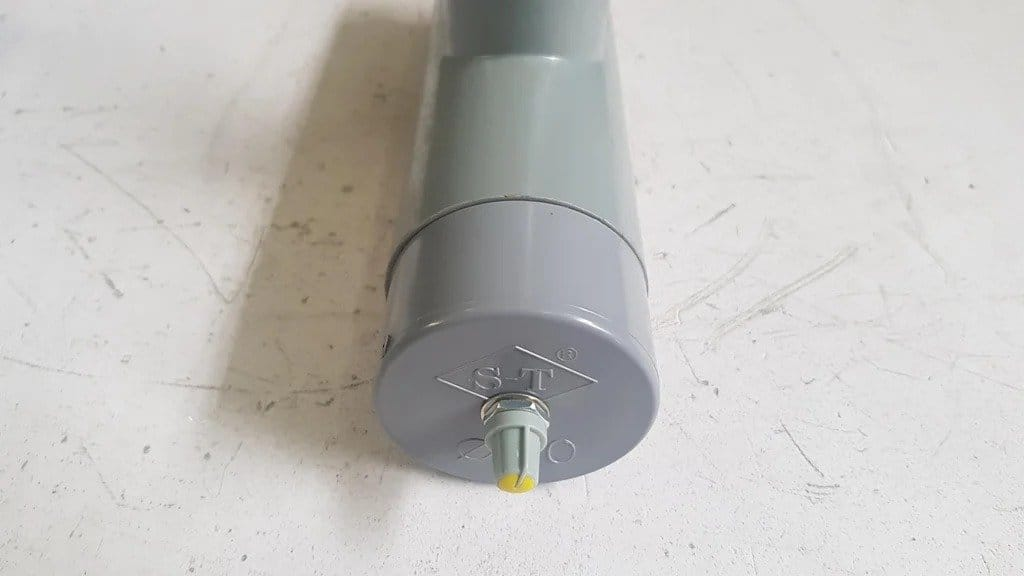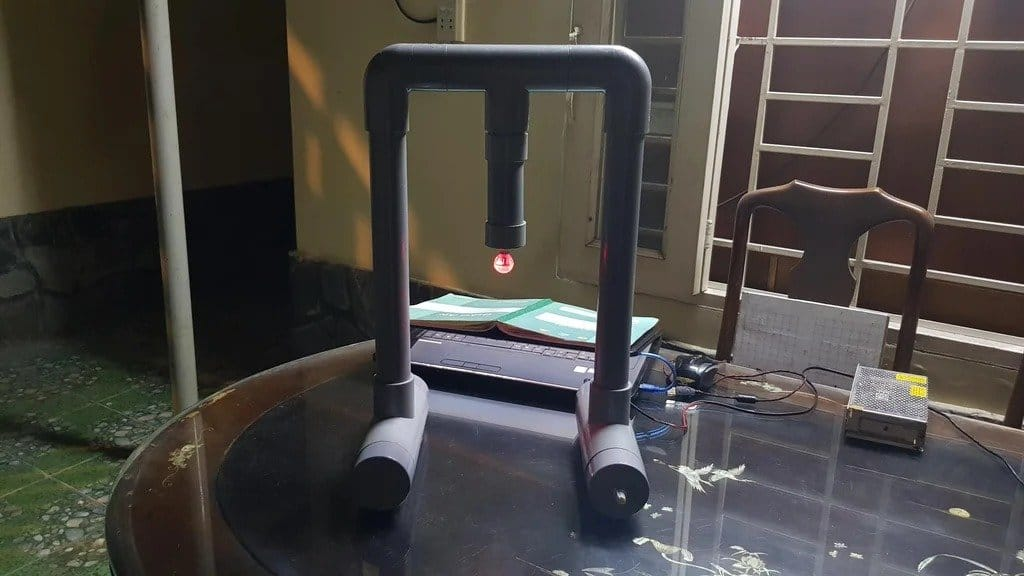He also tested other loads such as bolts. If the bolt is heavier than the LED ball, you need to decrease the setting from 330 to 320.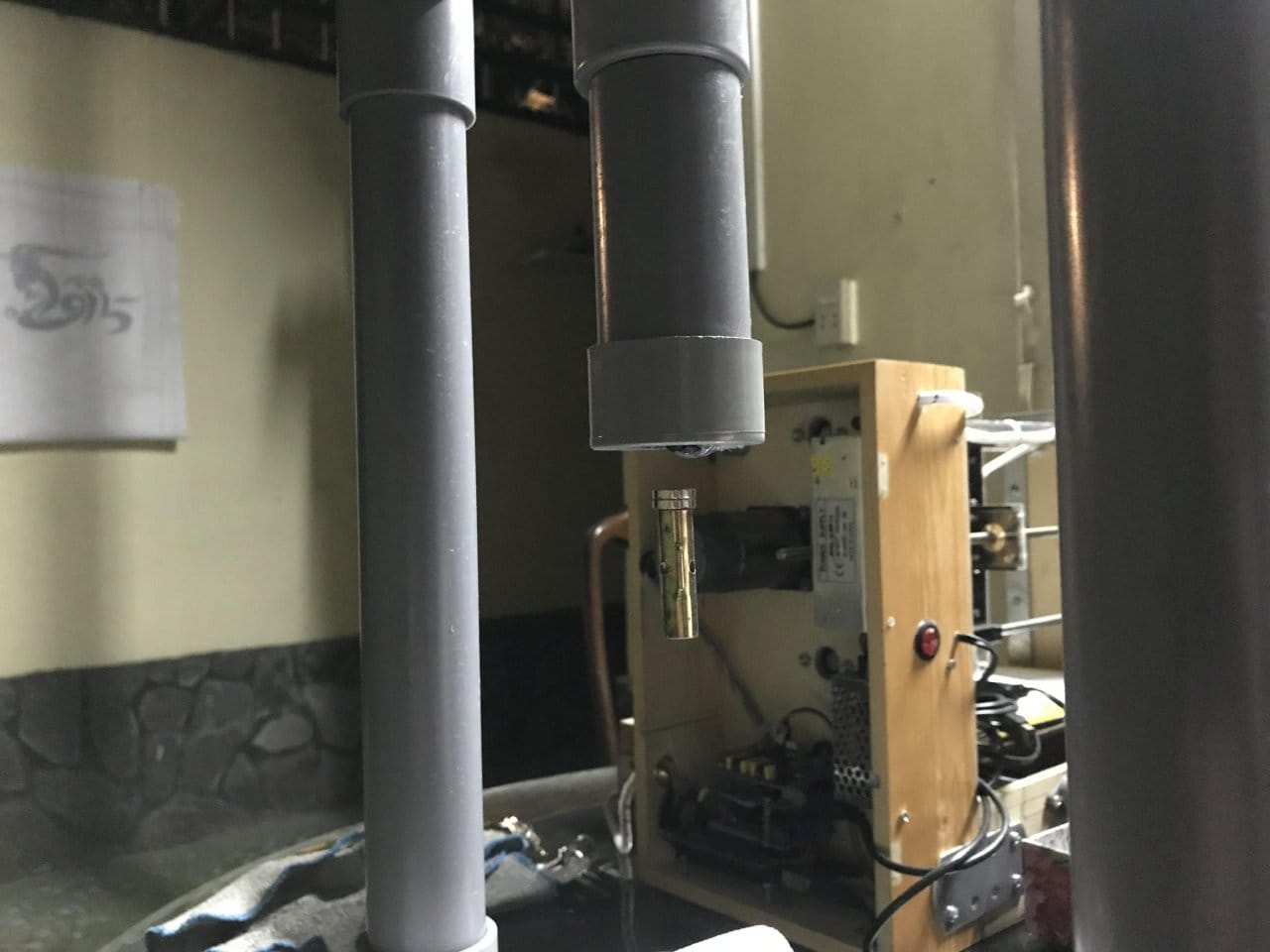< center>

Source:

usamodelkina.ru

Check Also
Close